CLAT  >  HCF and LCM Numbers

# HCF and LCM Numbers - Notes | Study Quantitative Techniques for CLAT - CLAT

 Table of contentsFactors and MultiplesPrime factorizationPrime factorization method to find the LCMProperties of HCF and LCM of given numbers:1 Crore+ students have signed up on EduRev. Have you?

Factors and Multiples

The factor is a divisor that does not give a remainder. A number has only a few factors, whereas it has unlimited multiples.

Example 42 = 7 x 6 = 7 x 3 x 2

7 and 6 are two factors of 42 whereas 42 is a multiple of 7 and is also a multiple of 6. 42 is a multiple of 2 and 3.

40 = 8 x 5

Also 4 x 5 x 2 = 40

So 40 is a multiple of 4, 5 and 2.

Multiples of 3 are 3, 6, 9, 12, 15, 18 ---------

Multiples of 4 are 4, 8, 12, 16, 20, 24 ------

Multiples of 5 are 5, 10, 15, 20, 25, 30 ------

Multiples of 6 are 6, 12, 18, 24, 30, 36 ------ and so on.

Properties of multiples:

(1) There can be unlimited number of multiples of a number

(2) Every number can be written as: the number x 1 i.e. every number is a multiple of 1

(3) Every number is a multiple of itself.

Factors: When we multiply any two or more numbers, we get a product. So the product is a multiple of the multiplied numbers. Each of the numbers multiplied is a factor of the product.

1 is a factor of every number. Every number is also a factor of itself. A number is a factor of a bigger number if the number divides the larger number without leaving any remainder.

Tests of divisibility of numbers:

To find whether a given number is divisible by another number, we perform actual division and test whether the remainder is zero or not. But the divisibility by 2, 3, 4, 5, 6, 8, 9, 10 and 11 can be performed simply by examining the digits of the given number.

(1) Test of divisibility by 2: A given number is divisible by 2 if its number at the unit place is 0 or divisible by 2.

Example: Each of the numbers 46, 926, 1022, 30954, 520 is divisible by 2.

(2) Test of divisibility by 3: A given number is divisible by 3 if the sum of its digits is divisible by 3

Example 1: Consider the number 32592

The sum of its digits is 3 + 2 + 5 + 9 + 2 = 21 which is divisible by 3. Therefore 32592 is divisible by 3

Example 2: Consider the number 46252

The sum of its digits is 4 + 6 + 2 + 5 + 2 = 19 which is not divisible by 3. So the no. 46252 is not divisible by 3

(3) Test of divisibility by 4: A number is divisible by 4 if the number formed by its digits in the tens and ones places is divisible by 4.

Example 1: Consider the number 97832

The number formed by tens and ones places is 32 which is divisible by 4

Therefore the number 97832 is divisible by 4

Example 2: Consider the number 60354

Te number formed by tens and ones places is 54 which is not divisible by 4

Therefore 60354 is not divisible by 4.

(4) Test of divisibility by 5: A number is divisible by 5 is the ones digit is 0 or 5

Example: Each of the numbers 85, 165, 710, 1330, 17825, 90165 is divisible by 5 and none of the numbers 16, 82, 183, 219, 579 is divisible by 5

(5) Test of divisibility by 10: A number is divisible by 10 if its unit digit is 0.

Example: Each of the numbers 20, 140, 2590 is divisible by 10 and none of the numbers 45, 167, 1296 is divisible by 10.

(6) Test of divisibility by 6: A number is divisible by 6 if it is divisible by both 2 and 3

Example 1: Consider the number 57612

Its unit digit being 2, it is divisible by 2

The sum of the digits is 21, so it is divisible by 3

So the no. 57612 is divisible by 6.

Example 2: Consider the number 92356.

The unit digit is 6, so it is divisible by 2

The total of the digits is 25 which is not divisible by 3, so the number is not divisible by 3

Therefore 92356 is not divisible by 6.

(7) Test of divisibility by 8: A number is divisible by 8 if the number formed by its digits in the hundreds, tens and one's places is divisible by 8

Example 1: Consider the number 67152

The number 152 is divisible by 8

So the number 67152 is divisible by 8

(8) Test of divisibility by 9: A number is divisible by 9 if the sum of its digits is divisible by 9

Example 1: Consider the number 63495

Sum of its digits is 6 + 3 + 4 + 9 + 5 = 27

Since 27 is divisible by 9 so the number 63495 is divisible by 9

(9) Test of divisibility by 11: A number is divisible by 11 if the difference of the sum of its digits in odd places and the sum of its digits in even places is either 0 or a multiple of 11.

Example 1: Consider the number 90728

Sum of its digits at odd places = 8 + 7 + 9 = 24

Sum of its digits at even places = 2 + 0 = 0

Difference of two sums = 24 – 2 = 22 which is divisible by 11

Therefore 90728 is divisible by 11

Example 2: Consider the number 643423

Sum of its digits at odd places = 3 + 4 + 4 = 11

Sum of its digits at even places = 2 + 3 + 6 = 11

Difference of two sums = 11 – 11 = 0

Therefore 643423 is divisible by 11.

Example 3: Consider the number 65844

Sum of its digits at odd places = 4 + 8 + 6 = 18

Sum of its digits at even places = 4 + 5 = 9

Difference of these sums = 18 – 9 = 9 which is not divisible by 11

Therefore the number 65844 is not divisible by 11.

General properties of divisibility

Property 1: If a number is divisible by another number, it must be divisible by each of the factors of that number.

Property 2: If a number is divisible by each of the two co-prime numbers, it must be divisible by their product.

Property 3: If a number is a factor of each of the two given numbers, then it must be a factor of their sum

Property 4: If a number is a factor of each of the two given numbers, then it must be a factor of their difference

Prime factorization

Prime factor: A factor of a given number is called a prime factor if this factor is a prime number.

Example 5 and 7 are prime factors of 35.

Prime factorization: To express a given number as a product of prime factors is called prime factorization of the given number.

Example 36 = 9 x 4

= 3 x 3 x 2 x 2

Note: Every composite number can be factorized into primes in only one way, the order of primes may vary

Twin primes: Two consecutive odd prime numbers are known as twin primes.

Pairs of twin primes between 1 and 100 are

(i) 3, 5  (ii) 5, 7  (iii) 11, 13         (iv) 17, 19         (v) 29, 31

(vi) 41, 43         (vii) 59, 61         (viii) 71, 73

Prime triplet: A set of three consective prime numbers differing by 2 is called a prime triplet.

e.g.   (3, 5, 7)

Perfect numbers: If the sum of all the factors of a number is two times the number, then the number is called a perfect number.

Example: (i) Factors of 6 are 1, 2, 3, 6 and their sum = 1 + 2 + 3 + 6 = 12 = 2 x 6

So 6 is a perfect number (ii) Factors of 28 are 1, 2, 4, 7, 14, 28 and their sum = 1 + 2 + 4 + 7 + 14 + 28 = 56 = 2 x 28

Therefore 28 is a perfect number.

Co-Primes: Two numbers are said to be co-primes if they do not have a common factor other than 1.

Example: (i) 2, 3  (ii) 3, 4  (iii) 4, 5  (iv) 4, 9  (v) 8, 15

Remember: Two prime numbers are always co-primes.

Two Co-primes need not be prime numbers.

Example: 6, 7 are co-prime but 6 is not a prime number

9, 10 are co-primes, while none of 9 and 10 is a prime number.

Highest Common factor (HCF): The greatest number which is a common factor of two or more given numbers is called their highest common factor or greatest common divisor or greatest common measure, written as HCF or GCD or GCM. HCF can be found by two different methods

1. By Prime Factorisation method:

(a) Find the prime factors of each of the given numbers.

(b) Find the common prime factors

(c) The product of these common prime factors is the HCF

Example 1: Find the HCF of 144 and 198

Step I    Prime factors of 144 are 2 x 2 x 2 x 2 x 3 x 3

Prime factors of 198 are 2 x 3 x 3 x 11

Step II  Common prime factors are 2, 3, 3

Step III Product of 2, 3, 3 is 2 x 3 x 3 = 18

Example 2: Find the HCF of 396 and 1080 by prime factorization method

Step I Prime factors of 396 are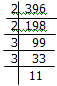Prime factors of 1080 are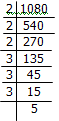Step II Common prime factors of 396 & 1080 are

2, 2, 3, 3

Step III Their product is 2 x 2 x 3 x 3 = 36.

Example 3: Find the HCF of 144, 180 and 192 by prime factorisation method.

Step I We have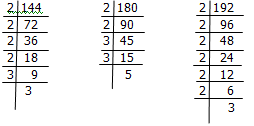Step II Common prime factors are

2, 2, 3,

Step III HCF is 2 x 2 x 3 = 12

HCF by division Method: Divide the greatest number by the smaller one. Next divide the divisor by the remainder. Go on repeating the process of dividing the proceeding divisor by the remainder last obtained till zero remainder is obtained. Then the last divisor is the required HCF.

Example1: Find the HCF of 513 and 783 by divison method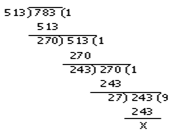Hence the HCF is 27.

HCF of more than two numbers (say three numbers)

Choose any two of the given numbers and find their HCF. Now the HCF of this HCF and the third number gives the HCF of the given three numbers.

If four numbers are given then the HCF of the HCF of three numbers and the fourth number will be the HCF of four numbers and so on.

Example 2: Find the greatest number which divides 285 and 1249, leaving remainder 9 and 7 respectively.

Solution: A number (greatest) is to be found out which when divides 285 leaves remainder 9 and when it divides 1249 leaves remainder 7. It means the greatest number which divides (285-9) and (1249-7) exactly is to be found out. So let us find out the HCF of 276 and 1242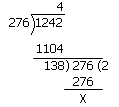Hence the reqd. number is 138

Check: If we divide 285 by 138 the remainder is 9 and on dividing 1249 by 138 the remainder is 7

Example 3: Reduce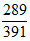to its lowest terms.

Solution: The given fraction can be reduced to its lowest terms if we divide the num. and den. by the highest common factor.

Let us find the HCF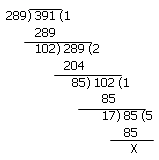HCF is 17

On dividing the numerator and denominator by 17

We get 17/23 as the fraction in its lowest terms.

Example 4: Three different containers contain 403 L, 434 L and 465 L of milk. Find the capacity of a container that will measure the milk of all the containers in an exact number of times.

Solution: Let us find the HCF of 403, 434 and 465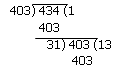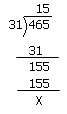So a container with a capacity of 31 L will measure the milk in the three given containers exactly.

Example 5: Find the largest number which divides 62, 132 and 237 to leave the same remainder in each case

Solution: In such types of questions where the same remainder is left in each case, find the difference of numbers from each other and then calculate the HCF of differences.

So Required number is the HCF of 132 – 62 = 70, 237 – 132 = 105 and 237 – 62 = 175

HCF of 70, 105 and 175 is 35

Example 6: Two numbers are in the ratio of 15: 11. If their HCF is 13, find the numbers.

Solution: Let the required numbers be 15x, 11x

Their HCF is given to be 13

HCF of 15x and 11x is x

So   x = 13

The numbers are 15x = 15 x 13 = 195 and 11x = 11 x 13 = 143

Example 7: The sum of two numbers is 216 and their HCF is 27. Find the numbers.

Solution: The HCF of two numbers is 27

So numbers will be of the type 27 a and 27 b

27a + 27b =216 or 27(a + b) = 216 or a + b = 8

A and b are two multiple prime numbers whose sum is 8

Such numbers are 1, 7 or 3, 5

So the numbers will be 27 x 1, 27 x 7

i.e.  278, 189

or 27 x 3,  27 x 5

i.e. 81,  135

So two pairs of numbers are possible 27, 189 and 81, 135

LCM = The lowest common multiple of two or more numbers in the smallest number which is a multiple of each of the numbers

Example: Let us find the LCM of 6 and 8

Solution: Multiples of 6 are 6, 12, 18, 24, 30, 36, 42, 48, 54, 60, 66, 72

Multiples of 8 are 8, 16, 24, 32, 40, 48, 56, 64, 72 -----

Common multiples of 6 and 8 are 24, 48, 72 -------

The lowest common multiple is 24 So LCM is 24

Prime factorization method to find the LCM

In order to find the LCM of two or more given numbers, we write the prime factors of each of the given numbers. Then the required LCM of these numbers is the product of all different prime factors of the numbers, using the greatest power of each common prime factor.

Example 1: Find the LCM of 24, 36 and 40 by prime factorization method.

Solution: Prime factors of 24 are 2, 2, 2, 3 = 23 x 3

Prime factors of 36 are 2, 2, 3, 3 = 22 x 32

Prime factors of 40 are 2, 2, 2, 5 = 23 x 51

So LCM f 24, 36 and 40 is 23 x 32 x 51 = 360

Example 2: Find the LCM of 112, 168 and 266 by prime factorization method.

Solution: Prime factors of 112 are 2 x 2 x 2 x 2 x 7 = 24 x 7

Prime factors of 168 are 2 x 2 x 2 x 3 x 7 = 23 x 3 x 7

Prime factors of 266 are 2 x 7 x 19         = 2 x 7 x 19

Therefore LCM of given numbers is the product of highest powers of all prime factors = 24 x 31 x 71 x 191 = 6384

Division Method to find LCM. In this method, we arrange the given numbers in a line, in any order. We divide these numbers by a number that divides exactly at least two of the given numbers and carry forward the numbers which are not divisible. The process is repeated till no two of the given numbers are divisible by a common number. The product of the divisors and the undivided numbers is the required LCM of the given numbers.

Example 3: Find the LCM of 12, 15, 20, 27 & 36 by the division method.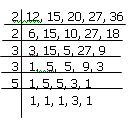So LCM of given numbers is 2 x 2 x 3 x 3 x 5 x 3 = 540

Properties of HCF and LCM of given numbers:

1) The HCF of a group of numbers is not greater than any of the numbers.

2) The HCF of two co-primes is 1.

3) The LCM of a group of numbers is not less than any of the given numbers.

4) The LCM of two co-primes is equal to their product.

5) The HCF of a group of numbers is always a factor of their LCM.

6) An important Property.

If two numbers are given then

The product of these two numbers = The product of their HCF & LCM

7) If a and b are two given numbers such that a is a factor of b then their HCF is a and LCM is b.

Example 3: The HCF of two numbers is 23 and their LCM is 1449, if one of the numbers is 161, find the other.

Solution: Another number is =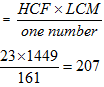Example 4: Find the LCM of 22, 54, 108, 135 and 198 by division method.

Solution: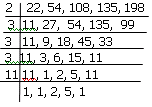So LCM = 2 x 3 x 3 x 11 x 5 x 2

= 5940

Example 5: Find the smallest number which when diminished by 3 is divisible by 21, 28, 36 and 45

Solution: We know that the smallest number divisible by 21, 28, 36, 45 is their LCM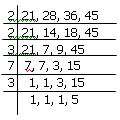LCM = 2 x 2 x 3 x 7 x 3 x 5 = 1260

The required no. is 1260 + 3 = 1263.

Example 6: Find the smallest number which when increased by 5 is divisible exactly by 15, 20, 24, 32, 36.

Solution: We know that the smallest number divisible by 15, 20, 24, 32, 36 is their LCM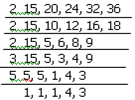LCM is = 2 x 2 x 2 x 3 x 5 x 4 x 3

= 1440

So the number is 1440 – 5 = 1435

The document HCF and LCM Numbers - Notes | Study Quantitative Techniques for CLAT - CLAT is a part of the CLAT Course Quantitative Techniques for CLAT.
All you need of CLAT at this link: CLAT

## Quantitative Techniques for CLAT

56 videos|35 docs|91 tests

## Quantitative Techniques for CLAT

56 videos|35 docs|91 tests

Track your progress, build streaks, highlight & save important lessons and more!

,

,

,

,

,

,

,

,

,

,

,

,

,

,

,

,

,

,

,

,

,

;